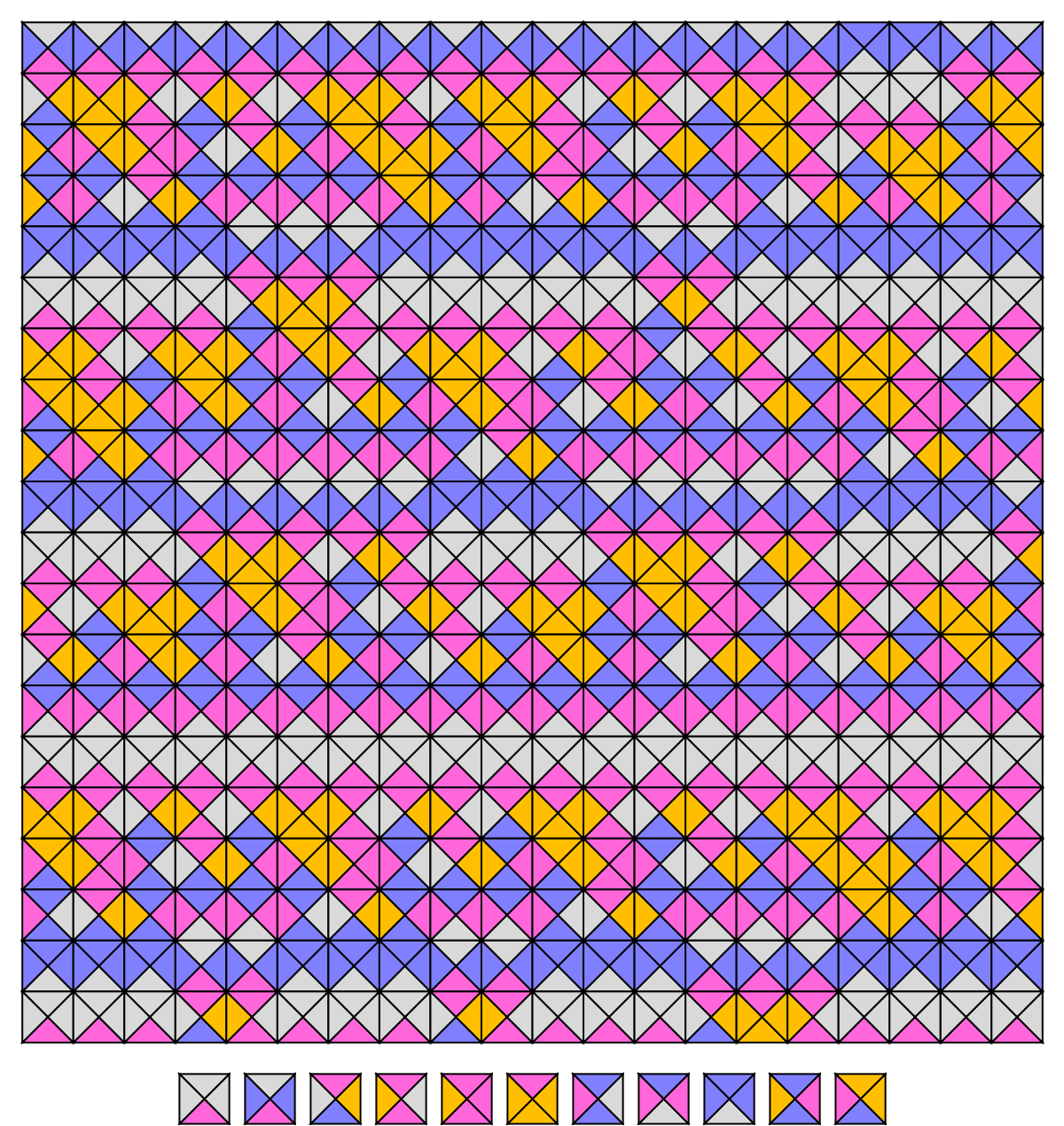Function Repository Resource:

# GenerateWangTiling

Generate a tiling pattern from a set of Wang tiles

 ResourceFunction["GenerateWangTiling"][tiles,{},n] covers an n× n array with edge-matching Wang tiles. ResourceFunction["GenerateWangTiling"][tiles,{},{m,n}] covers an m× n array instead. ResourceFunction["GenerateWangTiling"][tiles,{},size,All] lists all array coverings consistent with edge matching rules of the tiles. ResourceFunction["GenerateWangTiling"][tiles,{},size,count] lists multiple coverings, up to integer count of them. ResourceFunction["GenerateWangTiling"][tiles,seed,size,count] forces the seed tile to occur near the center of the pattern.

## Details

Generally, a Wang tile is a square whose South, East, North, and West edges are labeled with a matching color.
Two adjacent Wang tiles match on either North / South or East / West boundary when they have the same matching color on their shared edge.
Often matching colors are represented by numbers or more generally symbols. Here we prefer to use non-negative integers.
Input tiles should list a set of Wang tiles, each encoded as list of exactly four non-negative integers.
The four matching symbols are assumed to have a counter-clockwise order on square edges: SENW.
Here we call any rectangular array of Wang tiles a tiling pattern.
An “admissible” tiling pattern is one whose shared edges also share the same matching symbols.
ResourceFunction["GenerateWangTiling"] assumes that possible edge values in a tiling pattern are bounded above by the maximum integer found in the set of tiles.
ResourceFunction["GenerateWangTiling"] takes one Option "Boundary". When set to "Periodic", ResourceFunction["GenerateWangTiling"] also requires edge matching across North-South and East-West edges (i.e. toroidal boundary conditions).

## Examples

### Basic Examples (2)

Generate part of a Wang tiling from a set of just one tile:

 In:=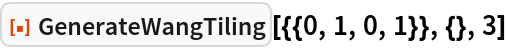Out=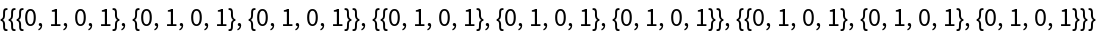Depict the 3×3 tiling as a Graphics object:

 In:=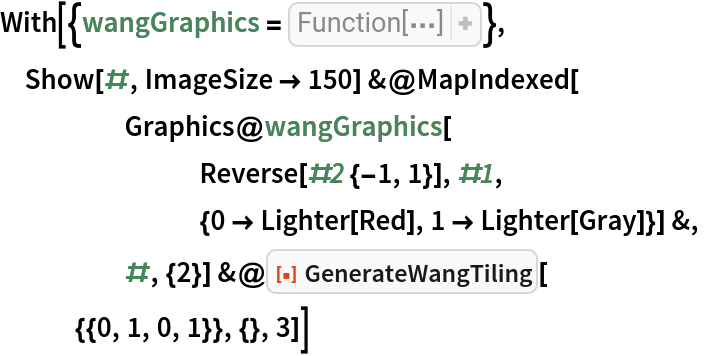Out=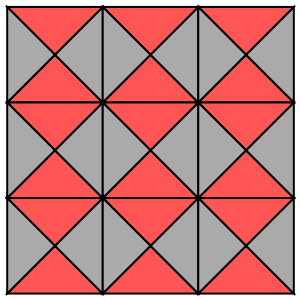Change the region size to 2×4:

 In:=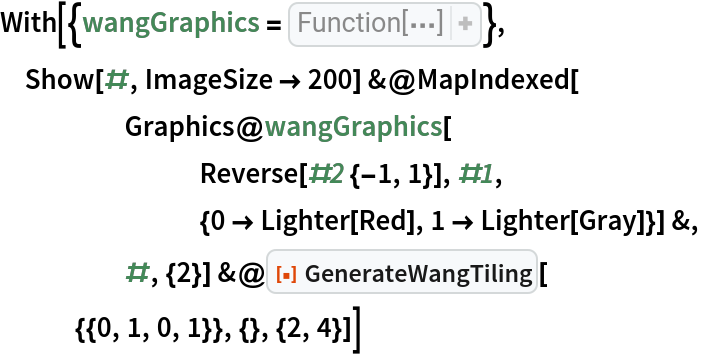Out=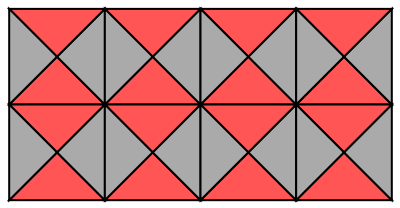Plot a periodic pattern by alternating between two Wang tiles:

 In:=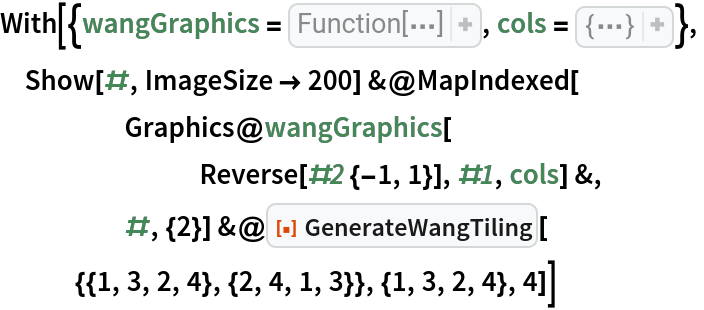Out=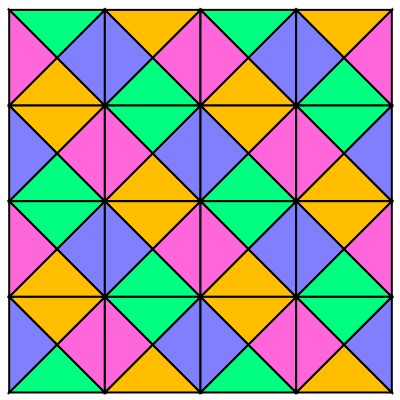Shift the pattern's offset by changing the seed tile:

 In:=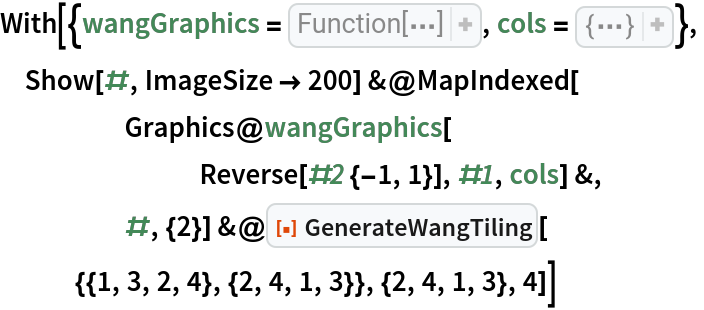Out=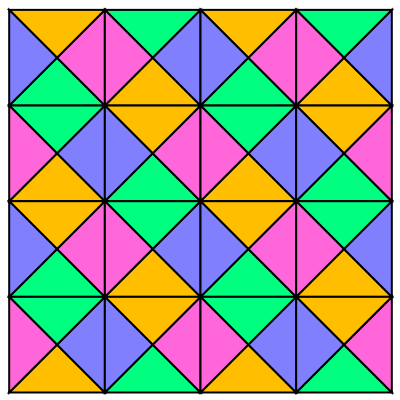Verify that this tiling pattern in four colors has only two translates:

 In:=Out=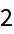### Scope (1)

GenerateWangTiling can get returns for bigger tile sets with more colors:

 In:=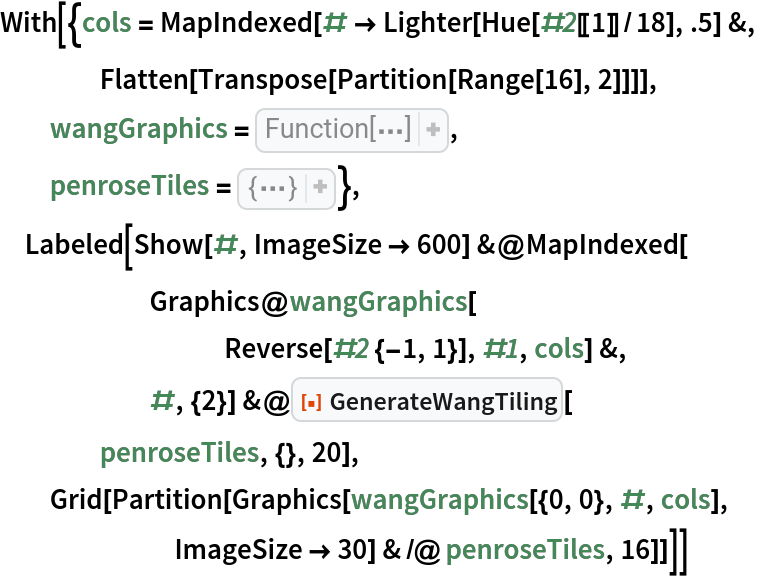Out=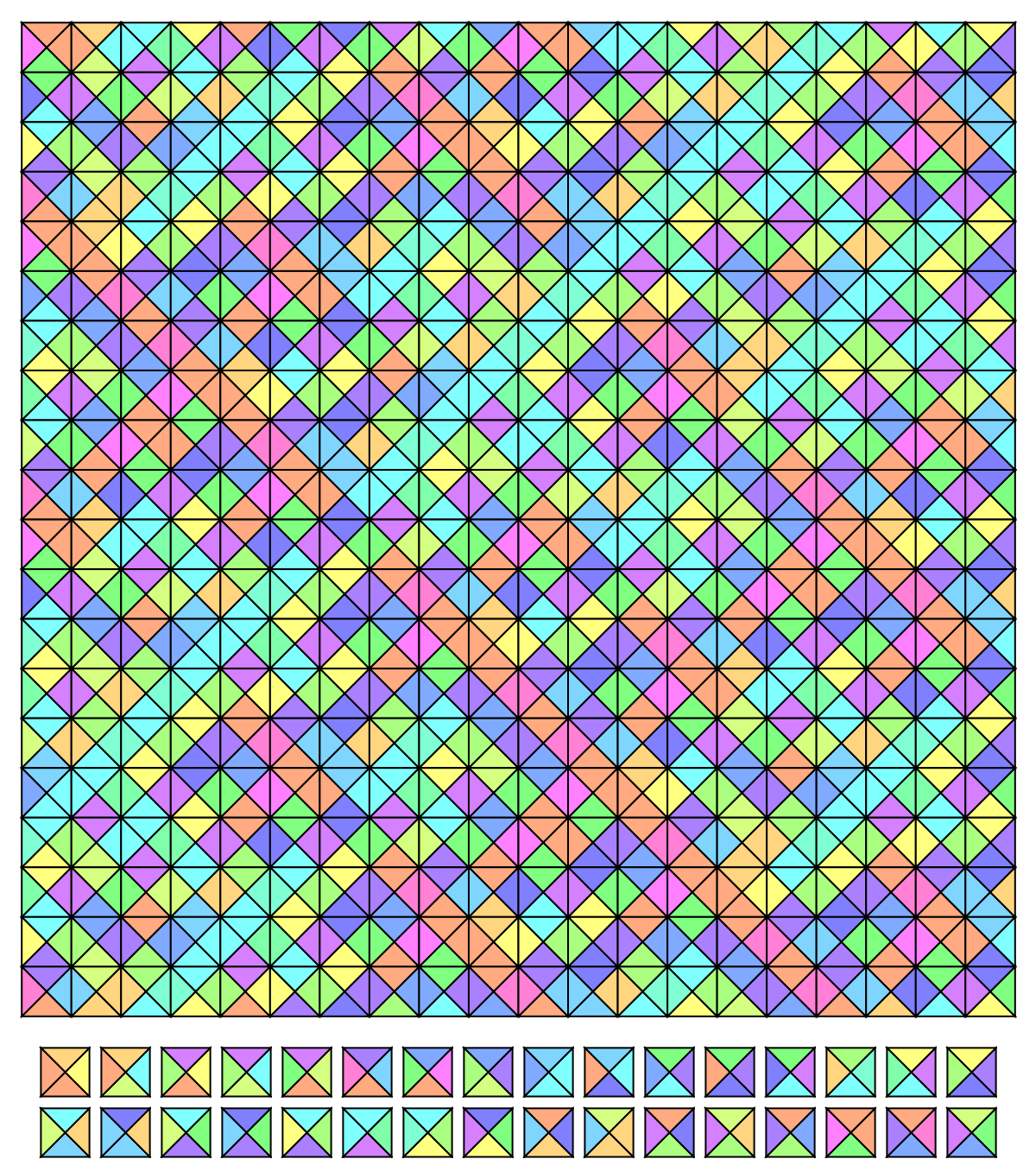### Options (1)

GenerateWangTiling can also check boundary conditions:

 In:=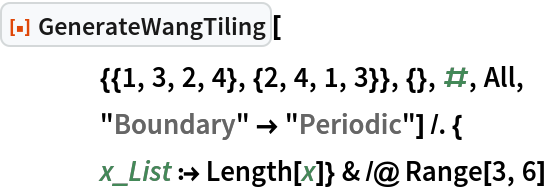Out=### Neat Examples (1)

Plot a section of the minimal-known aperiodic Wang tiling:

 In:=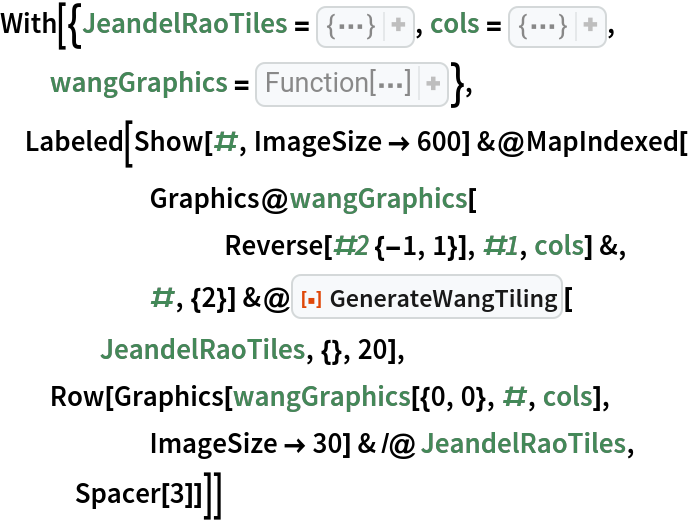Out=